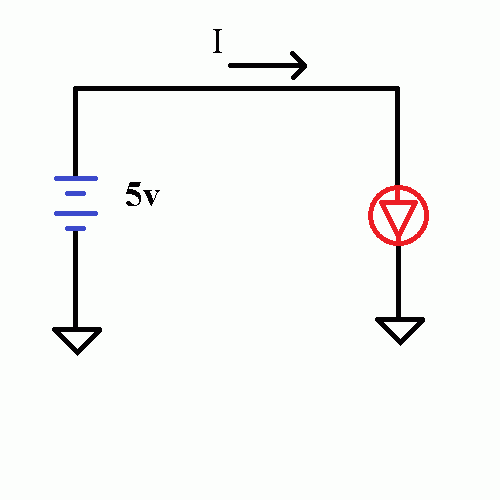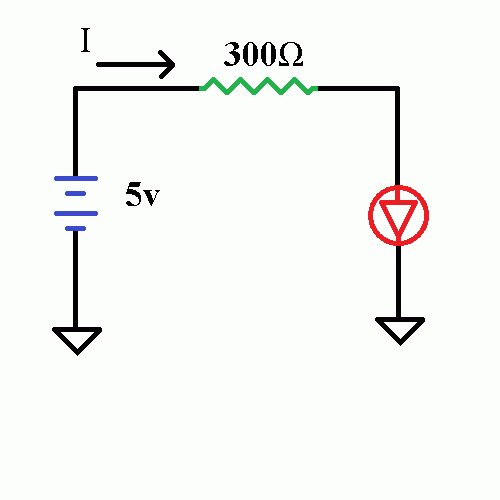What is a Current Limiting Resistor – Zero Ohm Electronics

What is a Current Limiting Resistor

Posted by Momen Odeh on

You might have read this term before, but what does it mean?

What is a current limiting resistor?

To answer this question, let me first ask you: how do you calculate current?
I = V/R
now, suppose you have a 5v source, and a 10K ohm resistor, you get:
I = 5/(10*10^3) = 0.0005A = 500uA
Again, suppose you have a 5v source but a 1 ohm resistor, you get:
I = 5/1 = 5A !!!!!that is really a huge amount of current
soooo, the less the resistance the more the current
and that is the answer! when the current is too much, you put a resistor to reduce (limit) it

Current Limiting Resistor Example

Have a Look at the circuit below:IVR explanation
what is the current (I) passing through the Red LED?
I = V/R
V = 5-2.2v= 2.8v (assuming a 2.2v forward voltage LED)
R =0.1 ohm (wire resistance, very small)
Therefore, I = 28A
Of course, the LED will burn and you will get an open circuit before you get to 0.1A :)
The solution is to add a "Current Limiting Resistor"Now, the current passing through the circuit is:
I = 2.8/300 = 0.009A = 9mA Good!

Uuse the formula:

R=V/0.02
0.02 indicates a current of 20mA, good for most 5mm LEDs, such as those LEDs we carry, if you are in doubt, read the datasheet of the particular LED you are using, aka RTFM (Read The Full Manual).If you face problems or if you have questions, feel free to use the comments section below.